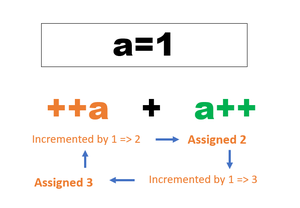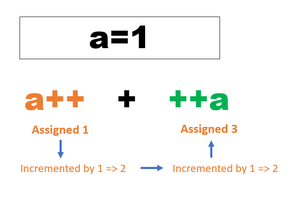Open In App

# Precedence of postfix ++ and prefix ++ in C/C++

In C/C++, precedence of Prefix ++ (or Prefix –) has same priority than dereference (*) operator, and precedence of Postfix ++ (or Postfix –) is higher than both Prefix ++ and *.  If p is a pointer then *p++ is equivalent to *(p++) and ++*p is equivalent to ++(*p) (both Prefix ++ and * are right associative).

Program 1:

For example, program 1 prints ‘h’ and program 2 prints ‘e’

## C++

 `// C++ program to explain the precedence of 'Prefix ++'` `#include ``using` `namespace` `std;` `int` `main()``{``    ``char` `arr[] = ``"geeksforgeeks"``;``    ``char``* p = arr;``    ``++*p;``    ``cout << *p;``    ``return` `0;``}` `// This code is contributed by sarajadhav12052009`

## C

 `// Program 1``#include``int` `main()``{``  ``char` `arr[] = ``"geeksforgeeks"``;``  ``char` `*p = arr;``  ``++*p;``  ``printf``(``" %c"``, *p);``  ``getchar``();``  ``return` `0;``}`

Output

`h`

Program 2:

## C++

 `// C++ Program to explain the precedence of 'Postfix ++'` `#include ` `using` `namespace` `std;` `int` `main()``{``    ``char` `arr[] = ``"geeksforgeeks"``;``    ``char``* p = arr;``    ``*p++;``    ``cout << *p;` `    ``return` `0;``}` `// This code is contributed by sarajadhav12052009`

## C

 `// Program 2``#include``int` `main()``{``  ``char` `arr[] = ``"geeksforgeeks"``;``  ``char` `*p = arr;``  ``*p++;``  ``printf``(``" %c"``, *p);``  ``getchar``();``  ``return` `0;``}`

Output

`e`

## C++

 `// C++ program that tells a person's last name` `#include ``using` `namespace` `std;` `int` `main()``{``  ``string fullName[] = {``"Joe"``, ``"Donaldson"``};``  ``string *ptr = fullName;``  ` `  ``cout << *ptr << ``"'s Last Name is "``;``  ``*ptr++;``  ``cout << *ptr << endl;``}` `// This code is contributed by sarajadhav12052009`

Output

`Joe's Last Name is Donaldson`

Here are some of the programs along with the concept which will help you in understanding the concept better.

When pre/post increment/decrement are used in statements with more than one operand then you have to take care of some rules.

Rule 1. When evaluating pre-increment value should be incremented first and will be assigned at the end of evaluating all the operands.

example :

## C++

 `#include ``using` `namespace` `std;` `int` `main() {` `    ``int` `a=1;``      ``cout<< ++a + a++ ;``}`

Now take some time and think of the output of the above program.

If You are thinking that the output should be 4 then you are wrong. The output will be 5

Here’s the explanation

1. pre-increment increments the value first and then assigns it. here ++a is incremented to 2 but is not assigned because a++ is remaining.

2. Then a++ is assigned 2 and then it is incremented to 3. Now the value of a is 3.

3. This final value will be assigned to ++a because pre-increment values are assigned at the last.

4. So the output will be 3+2= 5.If you think that you understood the concept then try the next example:

What do you think can be the answer to this example?

## C++

 `#include ``using` `namespace` `std;` `int` `main() {` `    ``int` `a=1;``      ``cout<

If you think what is the difference between this and the previous example then I must tell you that the difference is in the position of pre-increment and post-increment.  And the output will also be changed. You can run this program to check the output.

The output will be 4.

Here’s the explanation:

1. First the post-increment will be evaluated. It will be assigned 1 and then incremented to 2

2. then pre- increment will be incremented to 3 and then assigned to 3

So the sum of the assigned values is 1+3 = 4If you like this post then hit the like button and share it with your fellow mates and friends.

– This post has been improved by Triangleofcoding